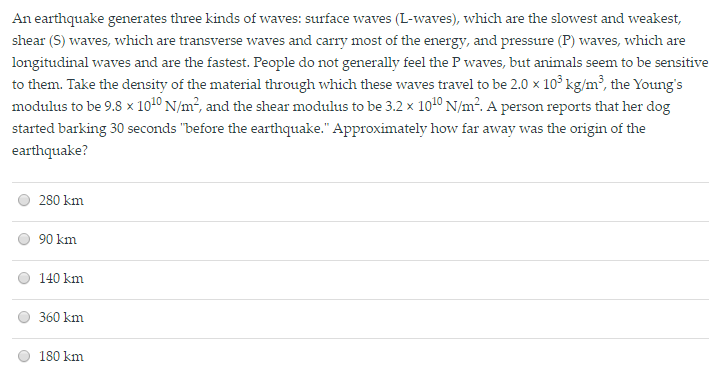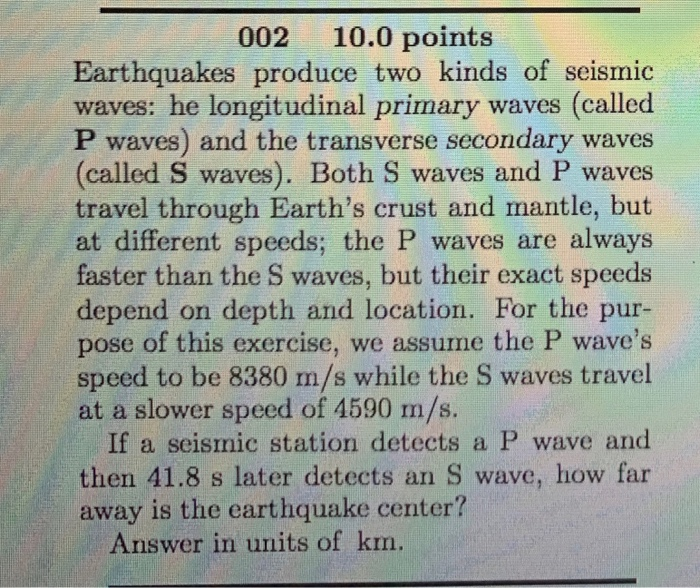Question

An earthquake generates three kinds of waves: surface waves (L-waves), which are the slowest and weakest,...An earthquake generates three kinds of waves: surface waves (L-waves), which are the slowest and weakest, shear (S) waves, which are transverse waves and earn- most of the energy, and pressure (P) waves, which are longitudinal waves and are the fastest. People do not generally feel the P waves, but animals seem to be sensitive to them. Take the density of the material through which these waves travel to be 2.0 times 10^3 kg/m^3, the Young's modulus to be 9.8 times 10^10 N/m^2, and the shear modulus to be 3.2 times 10^10 N/m^2. A person reports that her dog started barking 30 seconds "before the earthquake." Approximately how far away was the origin of the earthquake? 280 km 90 km 140 km 360 km 180 km

The speed of P waves is approximately 7 km/s, and that of S waves is about 4 km/s

x = ct
=> x = cp*tp = cs*ts

tp = cs/cp * ts

So,

tp - ts = ts(cs/cp - 1)

|tp - ts} = 30 s

= ts((4/7) -1)
= ts(0.43)

30 = ts(0.43)

=> ts = 69.76 s

So,
x = 4 km/s * 69.76
= 280 km (Ans)

Earn Coins

Coins can be redeemed for fabulous gifts.

Similar Homework Help Questions
• An earthquake generates three kinds of waves: surface waves (L-waves), which are the slowest and weakest,...

An earthquake generates three kinds of waves: surface waves (L-waves), which are the slowest and weakest, shear (S) waves, which are transverse waves and carry most of the energy, and pressure (P) waves, which are longitudinal waves and are the fastest. People do not generally feel the P waves, but animals seem to be sensitive to them. Take the density of the material through which these waves travel to be 2.0 × 103 kg/m3, the Young’s (bulk) modulus to be...

• how far is the dog from the earthquake's epicenter?

An earthquake generates three kinds of waves: surface waves (L waves), which are the slowest and weakest; shear (S) waves, which are transverse waves and carry most ofthe energy; and pressure (P) waves, which are longitudinal waves and travel the fastest. The speed of P waves is approximately 7.0 km/s, and that of S waves is about4.0 km/s. Animals seem to feel the P waves. If a dog senses the arrival of P waves and starts barking 34.7 s before...

• WAVE problem; Earthquakes produce two kinds of seismic waves: the longitudinal primary waves...?

Earthquakes produce two kinds of seismic waves: he longitudinal primary waves (called P waves) and the transverse secondary waves (called S waves). Both S waves and P waves travel through Earth’s crust and mantle, but at different speeds; the P waves are always faster than the S waves, but their exact speeds depend on depth and location. For the purpose of this exercise, we assume the P wave’s speed to be 8740 m/s while the S waves travel at a...

• 002 10.0 points Earthquakes produce two kinds of seismic waves: he longitudinal primary waves (called P...002 10.0 points Earthquakes produce two kinds of seismic waves: he longitudinal primary waves (called P waves) and the transverse secondary waves (called S waves). Both S waves and P waves travel through Earth's crust and mantle, but at different speeds; the P waves are always faster than the S waves, but their exact speeds depend on depth and location. For the pur- pose of this exercise, we assume the P wave's speed to be 8380 m/s while the S...

• The LlGO experiment, which historically detected gravitational waves for the first time in September 2015

The LlGO experiment, which historically detected gravitational waves for the first time in September 2015, uses a pair of highly sensitive Michelson interferometers. These have arms that are 4.00 km long and use powerful Nd-Yag lasers with 1064 nm wavelength. The beams traverse the arms both ways 280 times before recombining, which effectively lengthens the am length to 1120 km. The devices are found so that the beans destructively interfere when they recombine if no gravitational wave is present.The beam...